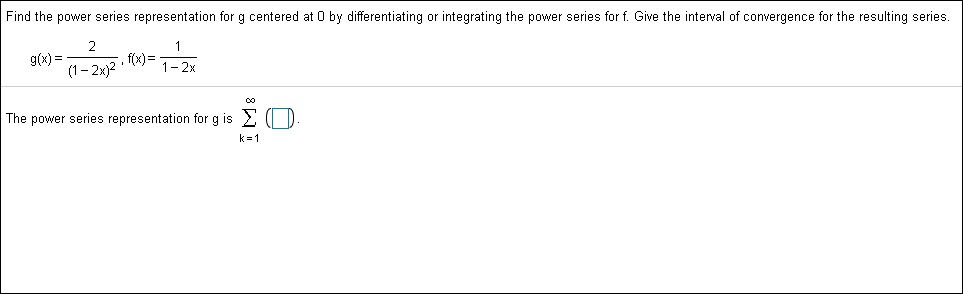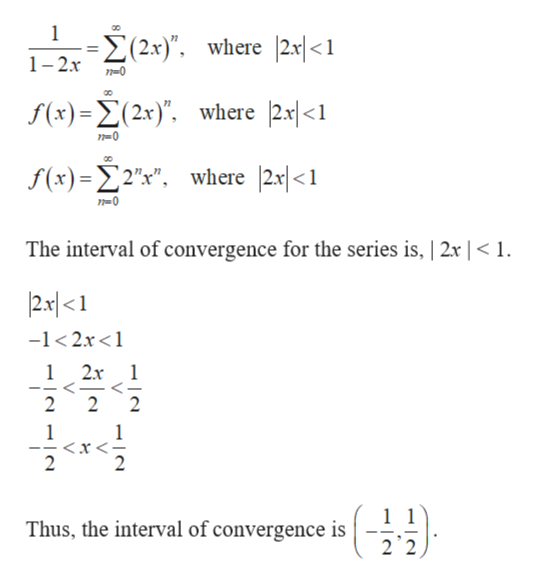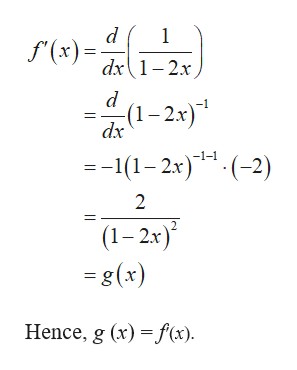# Find the power series representation for g centered at 0 by differentiating or integrating the power series for f. Give the interval of convergence for the resulting series2f(x)1-2xg(x)=1-2x)2The power series representation for g isk 1

Question
201 viewshelp_outlineImage TranscriptioncloseFind the power series representation for g centered at 0 by differentiating or integrating the power series for f. Give the interval of convergence for the resulting series 2 f(x)1-2x g(x)= 1-2x)2 The power series representation for g is k 1 fullscreen
check_circle

Step 1

Consider the geometric series,

Step 2

Substitute u = 2x in the above series to write the function f(x) in power series representation as follows.help_outlineImage Transcriptionclose1 -- Σ 2x), where 2y<1 1-2x 0 f(x)=(2x)" where 2s|<1 f(s) -Σ2ν", where 2<1 The interval of convergence for the series is, | 2x | < 1. 2x|<1 -1<2x 1 2x 1 <- 2 2 2 1 1 2 2 Thus, the interval of convergence is 2 2 fullscreen
Step 3

Differentiate the function f(x) with r...help_outlineImage Transcriptionclosed 1 f'(x)d1-2x. -(1-2x) dx -1-1 =-1(12x)(2) 2 (1-2x) = g(x) Hence, g (x) f(x) fullscreen

### Want to see the full answer?

See Solution

#### Want to see this answer and more?

Solutions are written by subject experts who are available 24/7. Questions are typically answered within 1 hour.*

See Solution
*Response times may vary by subject and question.
Tagged in

### Other